﻿ Design of Dynamic Model of Mechanisms with Serial Kinematic Structure

### Design of Dynamic Model of Mechanisms with Serial Kinematic Structure

Ivan Virgala, Michal Kelemen, Alexander Gmiterko, Tomáš Lipták

American Journal of Mechanical Engineering

## Design of Dynamic Model of Mechanisms with Serial Kinematic Structure

Ivan Virgala1,, Michal Kelemen1, Alexander Gmiterko1, Tomáš Lipták1

1Department of Mechatronics, Faculty of Mechanical Engineering / Technical University of Košice, Košice, Slovakia

### Abstract

The paper deals with modeling of dynamic model for mechanisms with serial kinematic structure. In the paper one of two basic methods for dynamic modeling is introduced, namely Euler – Lagrange method. The method is based on kinetic and potential energy of mechanism. The process of dynamic modeling analytically is very difficult task especially for kinematically redundant mechanisms. The aim of the paper is to show how can be the process of dynamic modeling automated by suitable algorithm programmed in software Matlab. The paper also show how the computing time increases in dependence on increasing number of mechanism links. This relation is expressed by polynomial function of 3rd order. At the end of the paper the demonstration of automatically generated inverse dynamic model is shown in cooperation with inverse kinematic model as well as trajectory planning task.

• Ivan Virgala, Michal Kelemen, Alexander Gmiterko, Tomáš Lipták. Design of Dynamic Model of Mechanisms with Serial Kinematic Structure. American Journal of Mechanical Engineering. Vol. 4, No. 7, 2016, pp 271-274. http://pubs.sciepub.com/ajme/4/7/8
• Virgala, Ivan, et al. "Design of Dynamic Model of Mechanisms with Serial Kinematic Structure." American Journal of Mechanical Engineering 4.7 (2016): 271-274.
• Virgala, I. , Kelemen, M. , Gmiterko, A. , & Lipták, T. (2016). Design of Dynamic Model of Mechanisms with Serial Kinematic Structure. American Journal of Mechanical Engineering, 4(7), 271-274.
• Virgala, Ivan, Michal Kelemen, Alexander Gmiterko, and Tomáš Lipták. "Design of Dynamic Model of Mechanisms with Serial Kinematic Structure." American Journal of Mechanical Engineering 4, no. 7 (2016): 271-274.

 Import into BibTeX Import into EndNote Import into RefMan Import into RefWorks

123
Prev Next

### 1. Introduction

The dynamic model explicitly describes the relationship between force / torques and motion. The equations of motion are important to consider in the design of robots, in simulation and animation of robot motion, and in the design of control algorithms . Many researches have been done in the area of dynamic modeling [2, 3, 4]. In the field of dynamics of mechanisms, the robotic community has especially focused on the problem of computational efficiency [5, 6].

In the paper dynamic model by Euler – Lagrange method is introduced. The aim of the paper is demonstration of automatic generation of dynamic model for redundant mechanisms with serial kinematic structure.

The paper is divided as follows. Section 2 deals with dynamic model using Euler – Lagrange method. In third section the process of automatic generation of dynamic model is introduced. This process is tested for several mechanisms with different numbers of links. Considering used PC parameters, the relation between computing time and number of links is established. Next section shows demonstration of automatic generated dynamic model on kinematically redundant mechanism. In the conclusion the results are discussed.

### 2. Dynamic Model

In general dynamic model knows two main task, namely forward and inverse. The aim of the forward dynamics is to compute the joint accelerations (and then velocities and positions) while there is given vector of generalized forces/ torques. The inverse dynamic model is method for computing of forces and torques based on known kinematic variables. In general, there are two basic methods by which are usually modelled dynamic models of multi body systems, namely Newton – Euler and Euler – Lagrange . Both methods are arise from different base. However, both of them gives the same result, which can be written as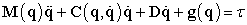(1)

where M is matrix of inertia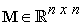, C is matrix of Coriolis and centrifugal forces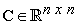, D is diagonal matrix considering friction forces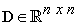, g is vector of gravity forces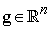,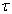is vector of torques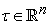and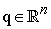is vector of generalized variables. Constant n represents number of DOF (degrees of freedom) of investigated mechanism.

In this section Euler – Lagrange formula is introduced. This method is based on kinetic and potential energy of mechanism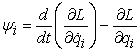(2)

where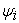represents non-conservative (extern and dissipative) generalized forces,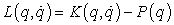is so called Lagrange function. Since potential energy is not dependent on velocity, equation (2) can be written as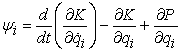(3)

Final kinetic and potential energy of investigated mechanism is given by sum of kinetic and potential energy of particular links of mechanism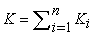(4)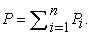(5)

Kinetic energy of i-th link is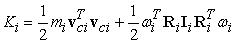(6)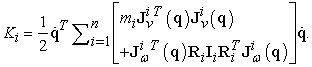(7)(8)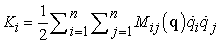(9)

where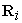is rotational matrix between fixed base and i-th link,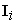is matrix of moments of inertia,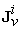and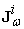are Jacobians for linear and revolute velocity.

Potential energy of i-th link is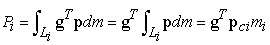(10)

where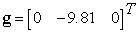. Total potential energy of mechanism equals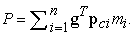(11)

Lagrange function is then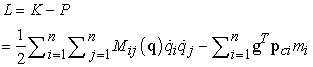(12)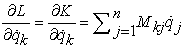(13)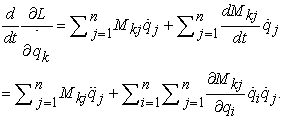(14)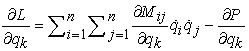(15)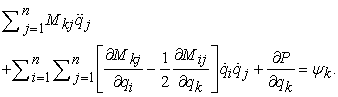(16)

By following substitution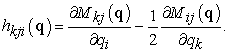(17)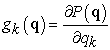(18)

one obtains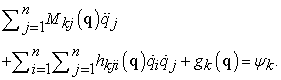(19)

Equation (19) can be rewritten in generalized form(20)

where matrix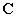can be expressed as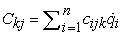(21)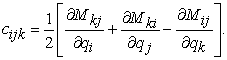(22)

Equation (22) represents so called Christoffel symbols.

### 3. Process of Automatic Generation of Dynamic model

Designing of dynamic model analytically can be very difficult task, in a lot of cases even impossible task. This fact is true especially in the cases of kinematically redundant mechanisms when difficulty of establishing of dynamic model terms is too high.

From this reason it is very appropriate to automate of whole process of dynamic model designing. Within this research the algorithm for automatic generation of dynamic model is introduced. The algorithm is designed for mechanisms with serial kinematic structure.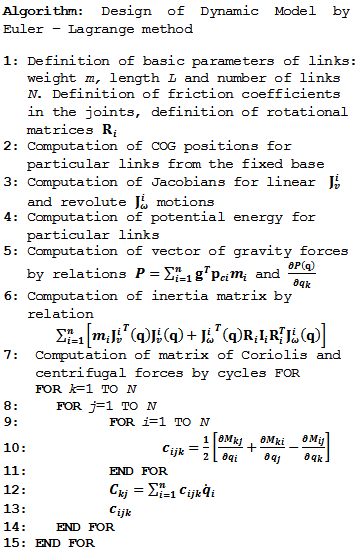By definition of basic parameters in the step 1 the algorithm gives on its output matrix of inertia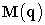, matrix of Coriolis and centrifugal forces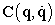and vector of gravity forces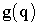. Designed algorithm is generalized and doesn´t matter from how many links the investigated mechanism consists of. The process of designing of dynamic model by this way is limited only by computing power of PC.

3.1. Testing of the Automatic Generation of Dynamic Model

Above introduced algorithm will be tested now for several cases. The aim of this section is to test how difficult this process is from the view of computing time. During the simulation the number of mechanism links will increase and our interest is in the computing time necessary for design of dynamic model.

The process is tested on PC with following parameters: Intel Core i3-4000M CPU 2.40 GHz, RAM 4,00 GB, OS: Windows 7 x64. The algorithm was written in Matlab 2013a. The simulations were performed ten times per each number of links and final computing time was determined as average value of these times. The results of the simulations can be seen in the Figure 1.Download asVeiw figureFigures index
Figure 1. Computing time necessary for generation of dynamic model

The final course can be also expressed by polynomial function of 3rd order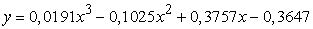(23)

Calculated reliability of equation (23) is R = 0,9998.

Let´s now consider kinematically hyper-redundant mechanism. According to equation (23) and considering above mentioned PC parameters, would be computing time for mechanism with 30 links 434,3563 s (roughly 7 min) and for mechanism with 100 links 18112,206 s (roughly 301 min). Of course, analytical solution for these cases is not humanly possible task.

### 4. Application of Designed Algorithm for Inverse Dynamic Model

The following section deals with application of automatic generation of dynamic model in cooperation with inverse kinematic model as well as trajectory planning task of redundant mechanism. Let´s consider mechanism with six links working in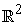. Since m=2 and n=6 the degree of redundancy is r=4.

The task is to find required torques of particular joints in order to move mechanism from its initial position to its goal position. The input parameter to the system is the point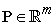to which the end-effector of investigated mechanism should be moved. At first, have to be used inverse kinematic model in order to determination of required final positions of joints. Subsequently have to be computed trajectory of particular links using polynomial of 5th order. The advantage of using polynomial of 5th order is obtaining of option to define initial and final angular accelerations of mechanism links. The output solution of trajectory planning is matrix of angular positions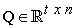, matrix of angular velocities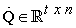and matrix of angular accelerations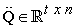, where n is number of links and t is number of geometrical points between initial and goal position of end-effector. The amount of this points is based on time, in which the end-effector should be moved from initial to goal position and also based on required time of simulation. The output of trajectory planner will be input to the model of inverse dynamics. The output of inverse dynamic model is vector of required torques.

By algorithm mentioned in section 3 can be generated dynamic model which is described by equation (20). Entire process described above is shown in the Figure 2.Download asVeiw figureFigures index
Figure 2. Algorithm for computation of required torques

In the Figure 3 are shown initial and goal configuration of investigated mechanism. Initial condition for all joints is 0 degree. The goal position is point inwith coordinates [1, 2]. Length of all links is the same, L = 1 m.Download asVeiw figureFigures index
Figure 3. Initial and goal configuration of investigated mechanism

For computation of inverse kinematic model was used damped least squares method. The result of inverse kinematics are generalized variables q. Detailed description of this computation is out of range of this paper.

In the Figure 4, Figure 5 and Figure 6 are shown required angular positions, angular velocities and angular accelerations, respectively. These course are results of trajectory planner which works with polynomial function of 5th order.Download asVeiw figureFigures index
Figure 4. Angular positionsDownload asVeiw figureFigures index
Figure 5. Angular velocitiesDownload asVeiw figureFigures index
Figure 6. Angular accelerations

During the simulation were assumed zero initial and goal velocities and accelerations.

Considering sequence of computing process from the Figure 2 the final course of required torques is shown in the Figure 7.Download asVeiw figureFigures index
Figure 7. Required torques

As can be seen from the figures the simulation last 60 s. The lower time of the simulation would be, the higher torques would be required.

### 5. Conclusion

In the paper has been dealt with Euler – Lagrange method for dynamic modeling purposes. The algorithm for automatic generation of dynamic model is introduced. The algorithm programmed in Matlab is tested on kinematically redundant mechanisms. Considering used PC parameters, the relation between increases number of links and computing time is established. At the end of the paper designed automatic process is demonstrated on the six-link mechanisms with assuming inverse kinematic model, trajectory planning and inverse dynamic model.

### Acknowledgements

The author would like to thank to Slovak Grant Agency – project VEGA 1/0872/16 and KEGA 048TUKE-4/2014.

### References

  Spong, M. W., Hutchinson, S., Vidyasagar, M.. Robot Modeling and Control, JOHN WILEY & SONS, INC., 2006.In article  R. Featherstone. “The Calculation of Robot Dynamics using Articulated-Body Inertias,” International Journal of Robotics Research, vol. 2, no. 1, pp. 13-30, 1983.In article View Article  J. Y. S. Luh, M. W. Walker and R. P. C. Paul. On-Line Computational Scheme for Mechanical Manipulators," Trans. ASME, J. Dynamic Sys- tems, Measurement & Control, vol. 102, no. 2, pp. 69-76, 1980.In article View Article  Walker, M. W., Orin, D. E., “Efficient Dynamic Computer Simulation of Robotic Mechanisms," Trans. ASME, J. Dynamic Systems, Measurement & Control, vol. 104, pp. 205-211, 1982.In article View Article  Featherstone, R., Orin, D.. Robot Dynamics: Equations and Algorithms, IEEE International Conference on Robotics & Automation, pp. 826-834, 2000.In article View Article  L. Sciavicco and B. Siciliano. Modelling and control of robot manipulators. Springer Verlag, 2000.In article View Article  Asada, H. H., Introduction to Robotics, Massachusetts Institute of Technology, 2005.In article  Lewis, F. L., Dawson, D. M., Abdallah, Ch. T.. Robot Manipulator Control: Theory and Practice (Automation and Control Engineering) 2nd edition, 2003.In article View Article